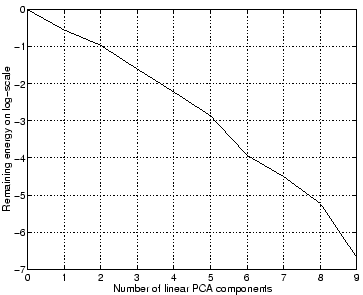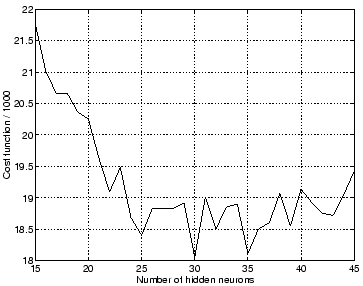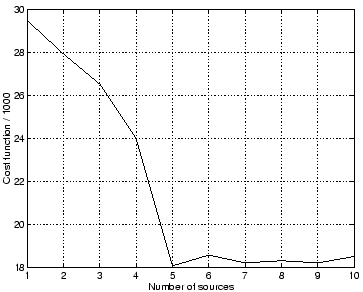Next: Non-Gaussian Sources. Up: Nonlinear Artificial Data Previous: Nonlinear Artificial Data

### Gaussian Sources.

The following experiments with nonlinear factor analysis algorithm demonstrate the ability of the network to prune away unused parts. The data was generated from five normally distributed sources through a nonlinear mapping. The mapping was generated by a randomly initialised MLP network having 20 hidden neurons and ten output neurons. Gaussian noise with standard deviation of 0.1 was added to the data. The nonlinearity for the hidden neurons was chosen to be the inverse hyperbolic sine, which means that the nonlinear factor analysis algorithm using MLP network with tanh-nonlinearities cannot use exactly the same weights.Figure 7 shows how much of the energy remains in the data when a number of linear PCA components are extracted. This measure is often used to deduce the linear dimension of the data. As the figure shows, there is no obvious turn in the curve and it would be impossible to deduce the nonlinear dimension.With the nonlinear factor analysis by MLP networks, not only the number of the sources but also the number of hidden neurons in the MLP network needs to be estimated. With the Bayesian approach this is not a problem, as is shown in Figs. 8 and 9. The cost function exhibits a broad minimum as a function of hidden neurons and a saturating minimum as a function of sources. The reason why the cost function saturates as a function of sources is that the network is able to effectively prune away unused sources. In the case of ten sources, for instance, the network actually uses only five of them.The pressure to prune away hidden neurons is not as big which can be seen in Fig. 10. A reliable sign of pruning is the amount of bits which the network uses for describing a variable. Recall that it was shown in Sect. 6.4.2 that the cost function can be interpreted as the description length of the data. The description length can also be computed for each variable separately and this is shown in Fig. 10. The MLP network had seven input neurons, i.e., seven sources, and 100 hidden neurons. The upper left plot shown clearly that the network effectively uses only five of the sources and very few bits are used to describe the other two sources. This is evident also from the first layer weight matrix A on the upper right plot, which shows the average description length of the weights leaving each input neuron.

The lower plot of Fig. 10 also shows the average description length of the weight matrix A, but now the average is taken row-wise and thus tells how many bits are used for describing the weights arriving to each hidden neuron. It appears that about six or seven hidden neurons have been pruned away, but the pruning is not as complete as in the case of sources. This is because for each source the network has to represent 1000 values, one for each observation vector, but for each hidden neuron the network only needs to represent five plus twenty (the effective number of inputs and outputs) values and there is thus much less pressure to prune away a hidden neuron.Next: Non-Gaussian Sources. Up: Nonlinear Artificial Data Previous: Nonlinear Artificial Data
Harri Lappalainen
2000-03-03